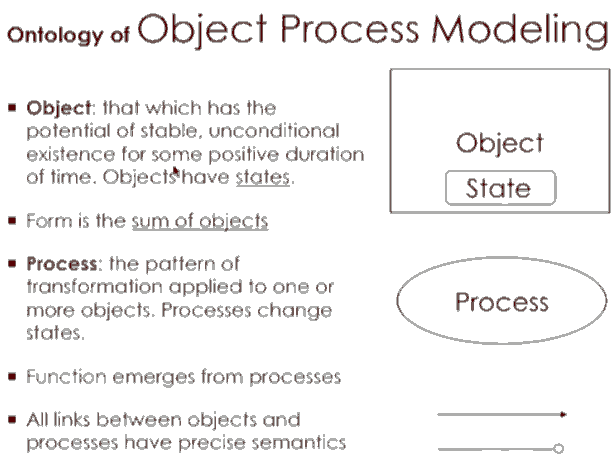# 一篇有关函数式编程的形象生动教程

18-11-12 banq

“硬件编程”是什么意思？我们可以看到专用集成电路（ASIC）中体现的“硬件编程” 。可以创建“编程”的电路，以便快速完成一件事，比如我的比特币下棋

FP中一个哲学上重要的概念是“函数相等 functionally equivalent”。

 ```# Ruby ZERO = -> (func) {    # does nothing   func } ```

 ```ONE = -> (func) {   # calls it once   # same as func.call()   func[];   func } TWO = -> (func) {   # calls it twice   func[]   func[]   func } ```

 ```HELLO = ->() { puts "hello" } # same as: ZERO.call(HELLO) ZERO[HELLO] # nothing displayed ONE[HELLO]  # one "hello" displayed TWO[HELLO]  # "hello" twice ```

 ```# convert number function into number object def to_integer(func)   # count how many times counter is called   n = 0   counter = ->() { n += 1 }   func[counter]   n end p to_integer(ZERO) # 0 p to_integer(ONE)  # 1 p to_integer(TWO)  # 2 ```

 ```ADD = -> (func1, func2) {   -> (f) { func1[func2[f]] } } sum = ADD[ZERO, ZERO] p to_integer(sum) # 0 sum = ADD[ZERO, ONE] p to_integer(sum) # 1 sum = ADD[ONE, ONE] p to_integer(sum) # 2 ```

sinaID75194
2018-11-13 15:40

sinaID75194
2018-11-13 16:09

banq
2018-11-13 16:33sinaID75194
2018-11-19 11:57